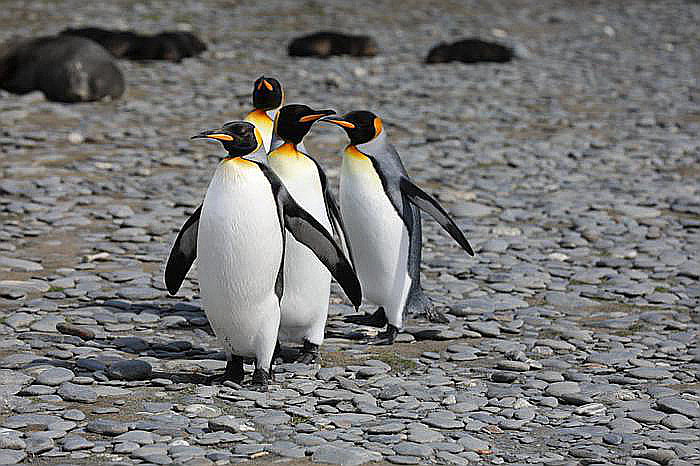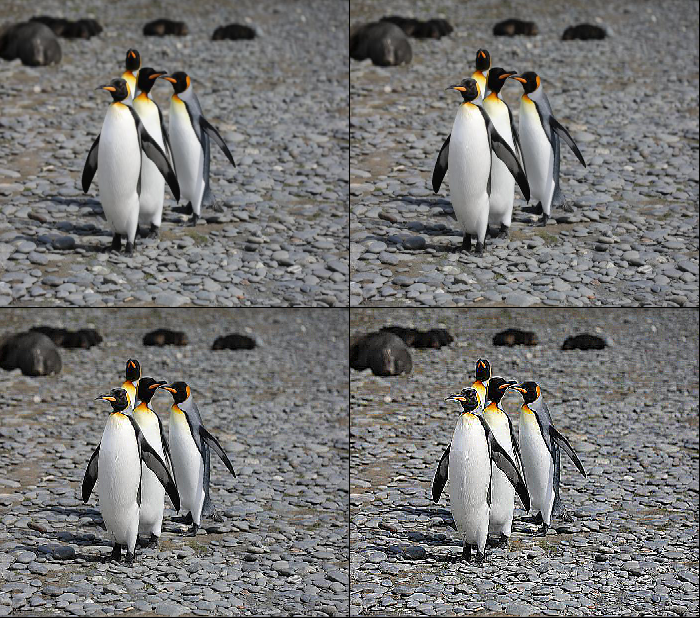# How to adjust the sharpness of an image in PyTorch?

To adjust the sharpness of an image, we apply adjust_sharpness(). It's one of the functional transforms provided by the torchvision.transforms module. adjust_sharpness() transformation accepts both PIL and tensor images.

A tensor image is a PyTorch tensor with shape [C, H, W], where C is number of channels, H is image height, and W is image width. This transform also accepts a batch of tensor images. If the image is neither a PIL image nor tensor image, then we first convert it to a tensor image and then apply the adjust_sharpness(). The sharpness should be any non-negative number.

### Syntax

torchvision.transforms.functional.adjust_sharpness(img, sharpness_factor)

### Parameters

• img – Image of which sharpness is to be adjusted. It is a PIL image or torch tensor. It may be a single image or a batch of images.

• sharpness_factor – A non-negative number. 0 will give a blurred image, while 1 will give the original image.

## Output

• It returns the sharpness adjusted image.

### Steps

To adjust the sharpness of an image, one could follow the steps given below −

• Import the required libraries. In all the following examples, the required Python libraries are torch, Pillow, and torchvision. Make sure you have already installed them.

import torch
import torchvision
import torchvision.transforms.functional as F
from PIL import Image
• Read the input image. The input image is a PIL image or a torch tensor.

img = Image.open('penguins.jpg')

• Adjust the sharpness of the image with the desired sharpness factor.

img = F.adjust_sharpness(img, 5.0)
• Visualize the sharpness adjusted image.

img.show()


### Input Image

We will use the following image as the input file in the second example.## Example 1

The following Python program blurs the input image with randomly chosen Gaussian blur.

# Import the required libraries
import torch
import torchvision
import torchvision.transforms.functional as F
import torchvision.transforms as T

# read input image as torch tensor

# Convert the image tensor to PIL image
img_pil = T.ToPILImage()(img)

# display the sharpness adjusted image
img_pil.show()

## OutputThe above output is the blurred image of the original input image.

## Example 2

import torch
import torchvision
import torchvision.transforms.functional as F
import torchvision.transforms as T
from torchvision.utils import make_grid

# make a grid of 4 images
grid = make_grid([img1, img2, img3, img4], nrow=2)

# Convert it to PIL Image
grid_img = T.ToPILImage()(grid)

# display the grid images
grid_img.show()
grid_img

## Output